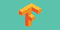# How to Build A ML model and Get Predictions using TensorFlow : (1/3)

Exploring the Dataset : To Predict Baby’s Weight.TensorFlow

Welcome. This is the 1st Tutorial from the series : How to Build A ML model and Get Predictions using TensorFlow.

In this series, we are going to use tf.estimator API.
Its a high-level TensorFlow API that greatly simplifies machine learning programming. It can be used for training, evaluation, prediction purposes in machine learning environment.

On Google Cloud Platform (GCP) , we can use Cloud ML Engine to train machine learning models in TensorFlow and other Python ML libraries (eg. scikit-learn).

Cloud ML Engine gives us a service for training and deploying…

# Building a Crawling Robot With Q Learning

This post is a part of our Introduction to Machine Learning course at Code Heroku.

In our Introduction to Reinforcement Learning post, we have seen how to model a Reinforcement Learning problem using Markov Decision Process (MDP).

In case of model based learning, we try to create a model for reinforcement learning problems using Markov Decision Process (MDP). We try to calculate all the possible probabilities associated with each state (and probabilities of getting rewards) and then extract a policy from it.

But, will calculating all the probabilities be computationally feasible for most real world problems? No, right? …

# Artificial Intelligence, Machine Learning and Deep Learning - Are they same?

One of the most common questions that beginners have when they start to learn Machine Learning is - “Are Artificial Intelligence(AI), Machine Learning(ML) and Deep Learning(DL) the same thing?”

And that’s a question which I personally hear a lot from so many people. So, I thought why not make a blog post to explain it?

## Artificial Intelligence (AI)

Artificial intelligence refers to the capability of a machine to imitate intelligent human behaviour. In other words, any machine that is able to perform tasks that would otherwise require human intelligence viz., …

# Introduction to Exploratory Data Analysis (EDA)

“Torture the data, and it will confess to anything.”

— Ronald Coase

If you are someone who is familiar with data science, I can confidently say that you must have realized the power of the above statement.

With proper use of data one could rule the entire world as well!

But, raw, unprocessed data isn’t of much use unless you derive insights from it.

Exploratory Data Analysis (EDA) is the process of visualizing and analyzing data to extract insights from it. …

# Introduction to Reinforcement Learning — Part 1

This post is a part of our Introduction to Machine Learning course at Code Heroku.

Till now in our Introduction to Machine Learning course, we have seen that a supervised machine learning algorithm takes in Features of a dataset (e.g., number of hours a student studies) and Labels (e.g., their score on an exam) as inputs and estimates a Function (model) which minimizes the difference between the expected and predicted results.

# How to get started with Machine Learning

Are you someone who is constantly trying to learn the concepts of machine learning and data science but don’t know from where to start?

If your answer is “YES”, then this is the right place for you.

Don’t worry, most people feel the same way as you when they first get introduced to machine learning. They have the enthusiasm to learn but, they get confused from where to start learning, which resources to follow and which prerequisites to learn at first.

So, we at Code Heroku, felt that it’s much needed for us to write an article about getting started…